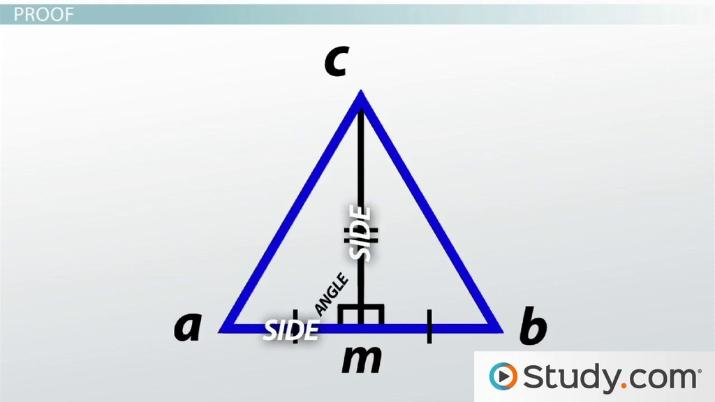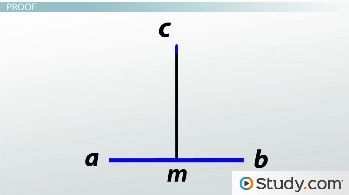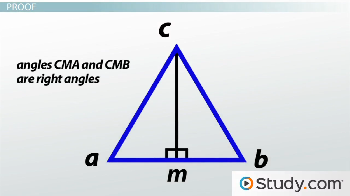Math Courses / Course / Chapter

# Perpendicular Bisector Theorem: Proof and Example

Lesson Transcript
Instructor: Jeff Calareso

Jeff teaches high school English, math and other subjects. He has a master's degree in writing and literature.

The perpendicular bisector theorem asserts that the point of perpendicular bisection of a segment is equidistant from either end. Examine the proofs, and the converse of this theorem through the provided examples. Updated: 09/30/2021

## Perpendicular Bisector Theorem

Let's talk perpendicular bisectors. These lines are immensely useful. Let's say you're an architect. I wanted to be an architect once. Then I realized it was less building cool models and more building regulations and codes. Anyway, let's completely ignore building codes here.

Here's some land. You're designing a skyscraper. The folks who hired you made two demands: it needs to go straight up and it needs to be in the middle of the land. Seems simple enough, right?

Say, do you know what you did? You made a perpendicular bisector. And it's not as simple as it seems. Consider the Leaning Tower of Pisa. Maybe it bisects the plots of land. But it definitely isn't perpendicular. Granted, who would visit the perpendicular tower of Pisa? These folks definitely ignored some building codes.

Anyway, perpendicular bisectors come with their very own theorem. The perpendicular bisector theorem states that if a point is on the perpendicular bisector of a segment, then it is equidistant from the segment's endpoints. In other words, if we hanged laundry lines from any floor of our tower, each floor would use the same length of laundry line to reach the ground. Okay, but I'm guessing the neighbors might complain about all the underwear hanging outside our tower.An error occurred trying to load this video.

Try refreshing the page, or contact customer support.

Coming up next: Angle Bisector Theorem: Proof and Example

### You're on a roll. Keep up the good work!

Replay
Your next lesson will play in 10 seconds
• 0:01 Perpendicular Bisector Theorem
• 1:21 Proof
• 3:45 Converse
• 6:04 Lesson Summary
Save Save

Want to watch this again later?

Timeline
Autoplay
Autoplay
Speed Speed

## Proof

But that's the theorem. Can we prove it? Here's a line, AB, and its perpendicular bisector. Let's label a point on the bisector C and the spot it hits AB as M.We're trying to prove that the lines we could draw from C to A and C to B are congruent. Adding those lines gives us more than just a place to hang our laundry, it gives us two triangles. That'll be important later.

Let's start by stating that CM is a perpendicular bisector of AB. We're given that. And, well, that's all we're given. But trust me, that's all we need.

Okay, now let's state that M is the midpoint of AB. That's part of the definition of a perpendicular bisector; it would be the bisector part of that definition. CM bisects AB, so the place where it hits AB is the exact middle. That means that AM is congruent to MB.

Why? Well, that's what a midpoint is - the spot that divides a line into two congruent segments. It also allows us to have pretty, symmetrical gardens on either side of our tower, or parking. Nothing beats ample parking.

Next, let's state that angles CMA and CMB are right angles. No leaning tower here. This is the other part of the definition of a perpendicular bisector; it's the perpendicular part, of course.Since those are right angles, we can state that angles CMA and CMB are congruent. All right angles are what? 90 degrees. And what's a 90 degree angle's relationship to another 90 degree angle? They're twins!

So we have congruent sides and congruent angles. All that came from one given statement. Not bad. And we're not done yet.

Let's state that CM is congruent to CM. File that under 'obvious statements.' Our tower equals itself. Officially, it's the reflexive property.

Okay, look what we have now: side-angle-side. That's not just a geometric palindrome, it's a postulate: SAS. That means that triangle CMA is congruent to triangle CMB.

And if triangle CMA is congruent to triangle CMB, and the corresponding parts of congruent triangles are congruent, which they are and we call CPCTC, then CA is congruent to CB. Two equal laundry lines! That's our proof!

## Converse

So the perpendicular bisector theorem is true. What about its converse? The converse of a statement is when you switch the hypothesis and conclusion. Instead of 'if p, then q,' the converse is 'if q, then p.'

To unlock this lesson you must be a Study.com Member.

### Register to view this lesson

Are you a student or a teacher?

Back

### Resources created by teachers for teachers

Over 30,000 video lessons & teaching resources‐all in one place.Video lessonsQuizzes & WorksheetsClassroom IntegrationLesson Plans

I would definitely recommend Study.com to my colleagues. It’s like a teacher waved a magic wand and did the work for me. I feel like it’s a lifeline.

Jennifer B.
TeacherCreate an account to start this course today
Used by over 30 million students worldwide